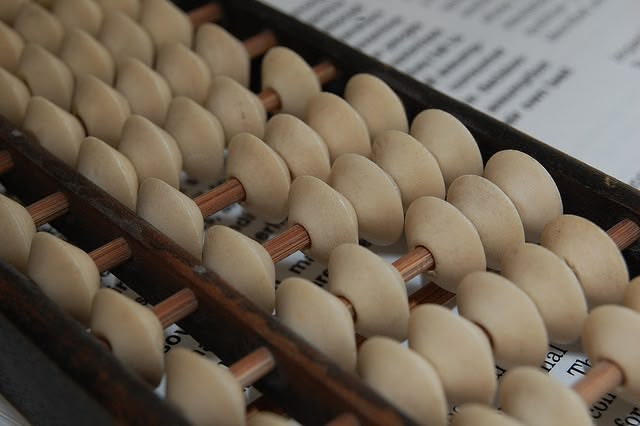My friends Tim and Megan over at Franktuary recently sent me a math puzzle and asked me why it worked. I thought it would be a fun little exercise to explain on the site. So here’s the puzzle:

<img align=”left” hspace=7px src=”/assets/timmegan.jpg”>

1. First pick the number of times a week that you would like to go out to eat (more than once but less than 10).
2. Multiply this number by two.
4. Multiply by fifty.
6. Subtract the four digit year you were born.

You should have a three digit number.
The first digit of this was your original number (i.e., how many times you want to go out to eat in a week).
The next two numbers are your age.
It’s said that this is the only year (2007) that this will work.

Here’s why this works:

First, you should notice that this puzzle has nothing to do with the number of times that you’d like to eat out every week. You could ask a person to randomly pick a number between 1 and 9 (including 1 and 9) and the puzzle would work out the same way.

If we go through step by step here’s why this works:

1. Let’s call the number that you initially pick x. It’s important to the puzzle that this number be between 1 and 9, including 1 and 9. You cannot pick 10. After we go through the explanation of the problem you should quiz yourself and ask why you cannot pick 10.

2. Multiply your number by 2. Now we have 2x.

3. Add 5 to this number. Now we have 2x + 5.

4. Multiply this number by 50. Now we have (50)(2x + 5). If we multiply this out we have 100x + 250.

5. Let’s assume that you’ve already had your birthday this year. According to the puzzle we should next add 1757 to our number. So we have (100x + 250) + 1757. Again, if we simplify we get 100x + 2007. Notice that the second half of this equation (after the plus sign) is the four digit year. Once we’re done with the puzzle you should ask yourself why we only add 1756 if we haven’t had our birthday yet this year.

6. At this point we’re supposed to subtract our four digit year of birth from our number. Since I was born in 1981 I’ll subtract this number. So our number is now (100x + 2007) - 1981. Simplifying, we get 100x + 26. This final equation is split into two parts. The second part (after the plus sign) will be your age. I am 26 years old. This is correct. The first part of the equation will be a multiple of 100, and will always be a three digit number. In other words, if you consider the three individual digits of this number, it will always be digit x followed by two digits of 0. So the number represented by the first part of this equation will basically be the number x00, if that makes sense. If you add 26 (or whatever your age is) to x00, you’ll always get x26.

The first digit will always be the number of times you’d like to eat out every week and the last two digits will be your age. And there’s the answer!

Here’s another two quiz questions for you:

1. Will this puzzle work for people of ALL ages, or just for specific ages?
2. How could you modify this puzzle so that it works in 2008 (or any other year for that matter)?

Well, hopefully that explains the puzzle. Let me know if you have any questions!